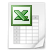# Free resourcesCalculation of mechanical characteristics of sections
The spreadsheet allows to calculate the mechanical characteristics of sections used in a lot of engineering problems.
It is possible to calculate the inertia and resistance parameters of the sections and the elastic/plastic characteristics.
A number of about 30 sections is reported.
File:
Calculation of mechanical characteristic
Microsoft Excel Table 2.1 MBForces on bolted connections
The spreadsheet allows to calculate the forces on the bolts in a bolted connection inserting the coordinates of each bolt composing the joint.
The external loads considered in the spreadsheet are the shear and tension forces, the bending and torque moments.
It calculates the center of the connection and the bolts shear forces along each axis. Specifying a bending pole position, it allows also to evaluate the tension force on each bolt.
These values can be used to verify the bolted joint, by means of specific bolt verification spreadsheet, as, for example, the one reported in the bolted connection category (“Dimensioning of Bolts”).
The spreadsheet is a demo version, so some sections are not available. For a description of the full version of the file see the bolted connection category.
File:
Forces on bolted connections v 2.1 - dem
Microsoft Excel Table 854.5 KBDimensioning of Bolts
The file is a demo version of the spreadsheet “Dimensioning of Bolts v 2.0”.
In this demo some sections of the full version are available, in particular:
- Calculus of bolt distances from edges
- Dimensioning of the friction working mode of a bolted connection. It takes into account the stiffness of the connected plates to evaluate the forces distribution between bolt and connected flanges and the tightening torque parameters. One or two shear planes can be selected.
For a description of the full version of the file see bolted connection category.
File:
Demo - Dimensioning of Bolts v 2.0.xls
Microsoft Excel Table 3.1 MBWelding verification
The file is a demo version of the spreadsheet “Welding Verification v 1.2”.
In this demo some sections of the full version are available, in particular: